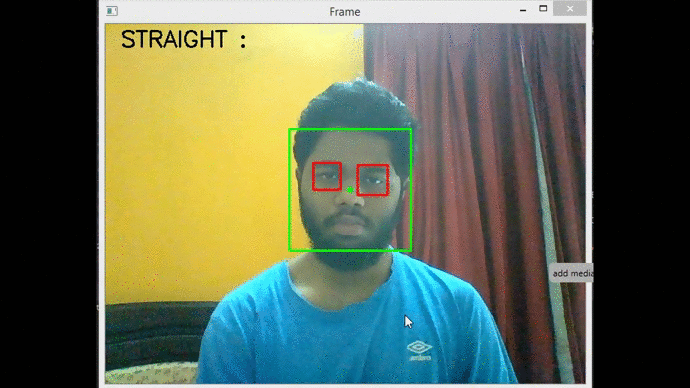# Determine The Face Tilt Using OpenCV – Python

• Difficulty Level : Medium
• Last Updated : 01 Oct, 2021

In this article, we are going to see how to determine the face tilt using OpenCV in Python.

To achieve this we will be using a popular computer vision library opencv-python. In this program with the help of the OpenCV library, we will detect faces in a live stream from a webcam or a video file and subsequently also determine the angle by how much the face is tilted.

## Algorithm:

• First, we detect the face in the webcam feed/video using the above-mentioned haarcascade classifier for the face and make a green color bounding box around it.
• Next, we detect the eyes using a similar haarcascade classifier trained on eyes and make a red color bounding box around each eye.
• In addition to making a box around each eye, we also identify and store the center of each box. Here, we are assuming that the center of the bounding box is the same as the center of the eye.
• For computing the angle of tilt we will assume that the line joining the centers of two eyes is perpendicular to the face.
• We have the coordinates of two centers in terms of (x,y) coordinates. The x-axis is the horizontal axis and y-axis is the vertical axis.
• When two points are given&, the anglewhich the line joining the two points makes with the x-axis can be obtained from geometry using the following expression:• In our case, the angle made by the line joining the centers of two eyes with the horizontal is computed. The positive angle indicates the right tilt and the negative angle indicates the left tilt.
• Provided a margin of error of 10 degrees (i.e, if the face tilts more than 10 degrees on either side the program will classify as right or left tilt).

## Python

 import cv2 as cvimport numpy as np  # 0 for webcam feed ; add "path to file"# for detection in video filecapture = cv.VideoCapture(0)face_cascade = cv.CascadeClassifier('haarcascade_frontalface_default.xml')eye_cascade = cv.CascadeClassifier("haarcascade_eye.xml") while True:    ret, frame = capture.read()    gray = cv.cvtColor(frame, cv.COLOR_BGR2GRAY)    faces = face_cascade.detectMultiScale(gray, 1.1, 5)    x, y, w, h = 0, 0, 0, 0    for (x, y, w, h) in faces:        cv.rectangle(frame, (x, y), (x + w, y + h), (0, 255, 0), 2)        cv.circle(frame, (x + int(w * 0.5), y +                          int(h * 0.5)), 4, (0, 255, 0), -1)    eyes = eye_cascade.detectMultiScale(gray[y:(y + h), x:(x + w)], 1.1, 4)    index = 0    eye_1 = [None, None, None, None]    eye_2 = [None, None, None, None]    for (ex, ey, ew, eh) in eyes:        if index == 0:            eye_1 = [ex, ey, ew, eh]        elif index == 1:            eye_2 = [ex, ey, ew, eh]        cv.rectangle(frame[y:(y + h), x:(x + w)], (ex, ey),                     (ex + ew, ey + eh), (0, 0, 255), 2)        index = index + 1    if (eye_1 is not None) and (eye_2 is not None):        if eye_1 < eye_2:            left_eye = eye_1            right_eye = eye_2        else:            left_eye = eye_2            right_eye = eye_1        left_eye_center = (            int(left_eye + (left_eye / 2)),          int(left_eye + (left_eye / 2)))                 right_eye_center = (            int(right_eye + (right_eye / 2)),          int(right_eye + (right_eye / 2)))                 left_eye_x = left_eye_center        left_eye_y = left_eye_center        right_eye_x = right_eye_center        right_eye_y = right_eye_center         delta_x = right_eye_x - left_eye_x        delta_y = right_eye_y - left_eye_y                 # Slope of line formula        angle = np.arctan(delta_y / delta_x)                  # Converting radians to degrees        angle = (angle * 180) / np.pi          # Provided a margin of error of 10 degrees        # (i.e, if the face tilts more than 10 degrees        # on either side the program will classify as right or left tilt)        if angle > 10:            cv.putText(frame, 'RIGHT TILT :' + str(int(angle))+' degrees',                       (20, 30), cv.FONT_HERSHEY_SIMPLEX, 1,                       (0, 0, 0), 2, cv.LINE_4)        elif angle < -10:            cv.putText(frame, 'LEFT TILT :' + str(int(angle))+' degrees',                       (20, 30), cv.FONT_HERSHEY_SIMPLEX, 1,                       (0, 0, 0), 2, cv.LINE_4)        else:            cv.putText(frame, 'STRAIGHT :', (20, 30),                       cv.FONT_HERSHEY_SIMPLEX, 1,                       (0, 0, 0), 2, cv.LINE_4)     cv.imshow('Frame', frame)     if cv.waitKey(1) & 0xFF == 27:        breakcapture.release()cv.destroyAllWindows()

Output:My Personal Notes arrow_drop_up